# Texas Go Math Grade 3 Module 6 Assessment Answer Key

Refer to our Texas Go Math Grade 3 Answer Key Pdf to score good marks in the exams. Test yourself by practicing the problems from Texas Go Math Grade 3 Module 6 Assessment Answer Key.

## Texas Go Math Grade 3 Module 6 Assessment Answer Key

Vocabulary

Choose the best term from the box.

Question 1.
The answer in a multiplication problem is called the ___. (p. 174)
Explanation:
a product is a number or a quantity obtained by multiplying two or more numbers together.

Question 2.
The numbers you multiply are called the ___.(p.174)
Factors
Explanation:
A factor is a number that divides the given number without any remainder.

Concepts and Skills

Write related addition and multiplication sentences. TEKS 3.4.D, 3.4.E

Question 3.
3 groups of 9
___ + ___ + ___ = ___
___ × ___ = _____
3 + 3 + 3 = 9
3 x 9 = 27
Explanation:
If you multiply by a given number and then added by the same number,
you will arrive at the same number you started with.

Question 4.
5 groups of 7
___ + ___ + ___ + ___ + ___ = ___
___ × ___ = _____
7 +7 + 7 + 7 + 7= 35
7 x 5 = 35
Explanation:
If you multiply by a given number and then added by the same number,
you will arrive at the same number you started with.

Draw jumps on the number line to show equal groups.
Find the product. TEKS 3.4.E, 3.4.K

Question 5.
6 groups of 3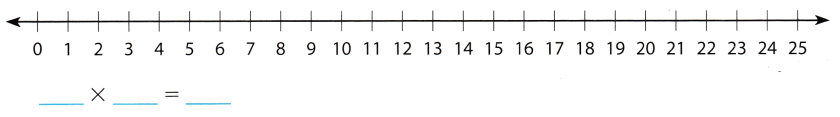Explanation:
If you multiply by a given number and then added by the same number,
you will arrive at the same number you started with.

Write a multiplication sentence for the model. Then use the Commutative Property of Multiplication to write a related multiplication sentence. TEKS 3.4.D, 3.4.E, 3.4.K, 3.5.B

Question 6.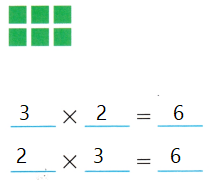Explanation:
If you multiply by a given number and then added by the same number,
you will arrive at the same number you started with.

Question 7.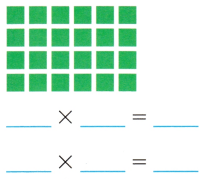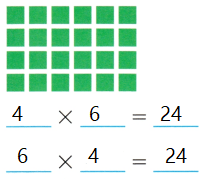Explanation:
If you multiply by a given number and then added by the same number,
you will arrive at the same number you started with.

Question 8.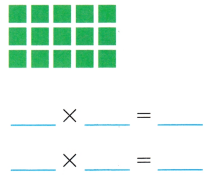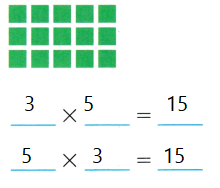Explanation:
If you multiply by a given number and then added by the same number,
you will arrive at the same number you started with.

Texas test Prep

Fill in the bubble for the correct answer choice.
You can use objects or models to solve.

Question 9.
Beth’s mother made some cookies. She put 4 cookies on each of 8 plates. Which equation can be used to find the number of cookies Beth’s mother put on the plates? TEKS 3.4.D, 3.4.E, 3.5.BAnswer: C and both are correct
Explanation:
Beth’s mother made some cookies. She put 4 cookies on each of 8 plates. So total is 8 x 4 = 32.
and  4 +4+4+4+4+4+4+4=32

Question 10.
Josh has 4 dogs. Each dog gets 2 dog biscuits every day. How many biscuits will Josh need for all of his dogs for Saturday and Sunday? TEKS 3.4.E, 3.4.K, 3.5.B
(A) 4
(B) 12
(C) 8
(D) 16
Explanation:
Josh has 4 dogs. Each dog gets 2 dog biscuits every day. So per dogs get 8 biscuits. Since, 4 x 2 = 8.
So 16 biscuits will Josh need for all of his dogs for Saturday and Sunday(2 days). Since 2 x 8 =16.

Question 11.
A unicycle has only 1 wheel. How many wheels are there on 9 unicycles? TEKS 3.4.K
(A) 11
(B) 9
(C) 1
(D) 10
Explanation:
A unicycle has only 1 wheel.9 wheels are there on 9 unicycles. Since 9 x 1 = 9

Question 12.
Lindsey needs 3 lengths of ribbon, each 5 inches long. Caleb needs 2 lengths of ribbon, each 6 inches long. How many inches of ribbon do they need altogether? TEKS 3.4.E, 3.4.K, 3.5.B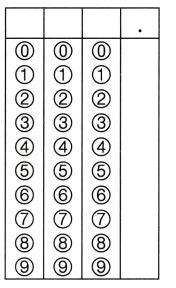Record your answer and fill in the bubbles on the grid. Be sure to use the correct place value.